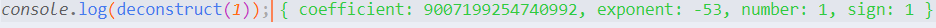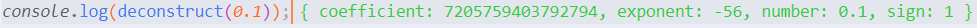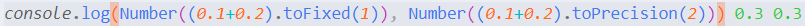# JS中数值类型的本质

126 0

## 一、JS中的数值类型

`0.5 <= 有效位数 < 1.0`

`数值 = 符号位 * 系数 * (2 ** 指数) `

## 二、JS中数值类型的本质

``````function deconstruct(number) {
let sign = 1;
let coefficient = number;
let exponent = 0;

//将符号位从系数中提取出来
if (coefficient < 0) {
coefficient = -coefficient;
sign = -1;
}

if (Number.isFinite(number) && number !== 0) {
//-1128 就是 Number.MIN_VALUE的指数减去有效位数再减去彩蛋位的结果
exponent = -1128;
let reduction = coefficient;
//将系数不断除以 2，直到趋近于 0 为止
while (reduction !== 0) {
//将除的次数与-1128相加到exponent
exponent += 1;
reduction /= 2;
}
//当指数为 0 的时候，可以认为数值是一个整数
//如果指数不为0，则通过校正系数来使其为 0
reduction = exponent;
while (reduction > 0) {
coefficient /= 2;
reduction -= 1;
}

while (reduction < 0) {
coefficient *= 2;
reduction += 1;
}
}
return {
sign,
coefficient,
exponent,
number
};
}
```````1 * 7205759403792794 * 2 ** -56 = 0.1000000000000000055511151231257827021181583404541015625`Number.toFixed()用于保留小数点具体位数进行四舍五入，参数默认为0，则不保留小数。Number.toPrecision()用于保留并四舍五入到指定的数字位数，默认全部保留，参数为0，则保留1位有效数字。注意，以上两个函数均返回string类型的值，所以在这里需要将其显示转换为number类型。

• 这篇文章还没有收到评论，赶紧来抢沙发吧~